# The Most Probable RadiusHydrogen Ground State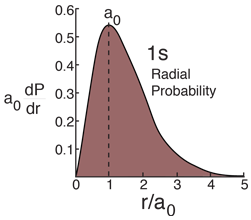The radial probability density for the hydrogen ground state is obtained by multiplying the square of the wavefunction by a spherical shell volume element.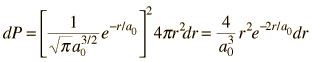It takes this comparatively simple form because the 1s state is spherically symmetric and no angular terms appear.

Dropping off the constant terms and taking the derivative with respect to r and setting it equal to zero gives the radius for maximum probability.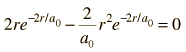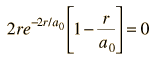which giveswhere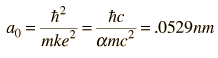The most probable radius is the ground state radius obtained from the Bohr theory. The Schrodinger equation confirms the first Bohr radius as the most probable radius but goes further to describe in detail the profile of probability for the electron radius.

Index

Schrodinger equation concepts

Hydrogen concepts

 HyperPhysics***** Quantum Physics R Nave
Go Back

# The Expectation Value for RadiusHydrogen Ground State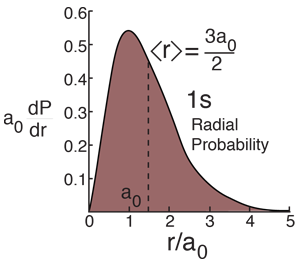The average or "expectation value" of the radius for the electron in the ground state of hydrogen is obtained from the integral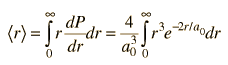This requires integration by parts. The solution is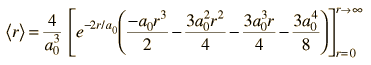All the terms containing r are zero, leaving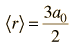It may seem a bit surprising that the average value of r is 1.5 x the first Bohr radius, which is the most probable value. The extended tail of the probability density accounts for the average being greater than the most probable value.